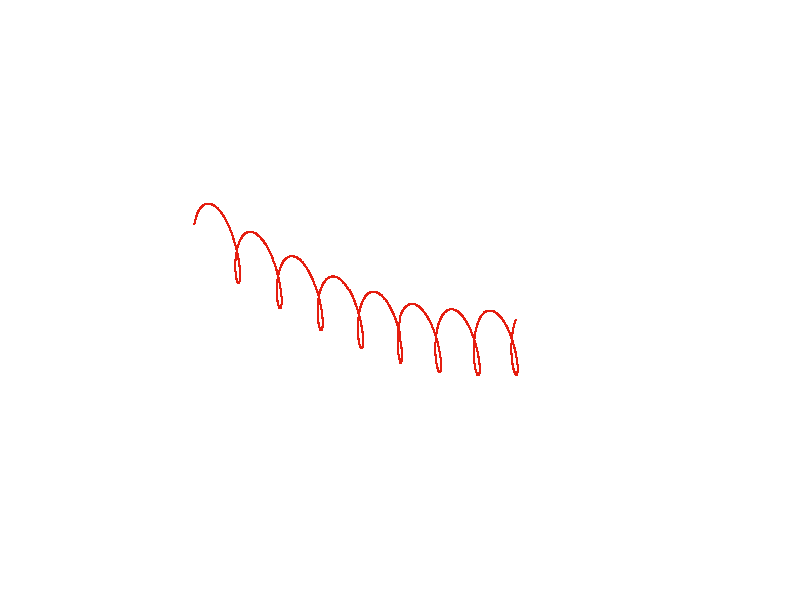# Orbiting the Moon

So far, we've only treated perturbations to an orbit which originated from the gravity field of Earth. However, this is not the only kind of perturbation there is - there are multiple other bodies in the solar system which each also excert gravitational pull on each other, and this corresponds to another class of perturbations.

In order to see how these perturbations can be simulated, let's first do something that has nothing to do at all with low Earth orbit and set up the simulation for an orbit around the Moon - while it orbits Earth.

## Defining celestial bodies

In order to simulate the gravitational effect of a body, we first need to tell the simulation two things - where to find it and how strong its gravity field is. Since the primary purpose of the software is the simulation of perturbations for Earth orbits, the position also needs to be specified in Earth-relative coordinates in terms of a geocentric ephemeris table. Such a table contains in the first column the simulation time in seconds, followed by the angular position in the sky in terms of a right ascension (converted to degrees) and a declination (in degrees), followed by the distance (in kilometers).

Specifying all objects in an Earth-centered system seems a bit awkward, but in fact there's plenty of data to be found because it's just the format which astronomers need - for instance Astropixels contains a large number of tables. A month worth of Sun and Moon movement data for Jan 2016 are included with with this software - if you just want to explore the effect of perturbations qualitatively you can use them, if you need to simulate a specific scenarios, you need to assemble your own tables.

The second important characteristic, the gravitational influence of the body, is specified in the form of the standard gravitational parameter GM (the product of gravitational constant and mass). This parameter (rather than the mass) is chosen because the product GM can be measured to far greater accuracy than either of its factors, and we in fact never need to know the mass of a body in the simulation.

The declaration of a celestial body then looks as follows:

 celestial_body name Moon ephemeris ephemerides/ephemeris_moon.dat GM 4.9048695e12

i.e. the body can have a name assigned, the emphemeris data is handed over via the file path (make sure this is correct for your directory structure!) and the GM parameter is directly given.

Since the ephemeris data has a finite coverage of times, the simulation including additional bodies can not be extended beyond the edge of the tables that are supplied.

## Celestial body coordinates

Now that we have the Moon defined, how can we initialize a spacecraft there? Even finding out where the Moon is in Earth-relative coordinates is not easy. But luckily we can make the software do it for us. Inserting

 list bodies initial

into the config file brings a list of the Moon's initial position and velocity. If we want to have a spacecraft orbiting, we can simply use these values and offset them by the radius of the orbit and the orbital speed - which for an orbit 1000 km above the Lunar surface gives
 state_vector x -402151000.0 y 23556100.0 z 10256000.0 vx 1230 vy -914.4 vz -303.605

Here the parameters have been chosen such that the spacecraft orbits in the xy plane (if you want a shortcut, the result of all the steps described here are also found in lunar_orbit.cfg) . In order to see how the orbit looks like, Earth-relative coordinates are unfortunately not really useful. This can be addressed by appending an '1' to the desired plot output

 plot2d file lunar_orbit_rel.dat x x1 y y1

which uses coordinates relative to the first declared celestial body. If you run this configuration, you should see a nice and nearly circular orbit.

## Orbiting the Moon

The same dynamics can also be plotted in 3d space in Earth-relative coordinates which yields the interesting wiggles in the line rotating around the orbit of the Moon itself as it moves around Earth.

 plot3d file lunar_orbit.dat x x y y z z

Unfortunately it's not as easy to determine the orbital elements (yet) - because they're computed Earth-relative still, and hence the output doesn't make too much sense. But the period of a 1000 km altitude orbit around the Moon ought to be about 12800 seconds, and you can try to verify that by varying the time up to which the simulation runs and see when the orbit closes.Multiple near-circular orbits around the Moon in Earth-relative coordinates.

Now, having convinced ourselves that the Moon is there in the simulation and exerts its gravitational influence, we can proceed to study how it perturbs orbits much closer to Earth.

Continue with Multibody perturbations.

Back to main index     Back to science     Back to LEO targeting

Created by Thorsten Renk 2018 - see the disclaimer, privacy statement and contact information.